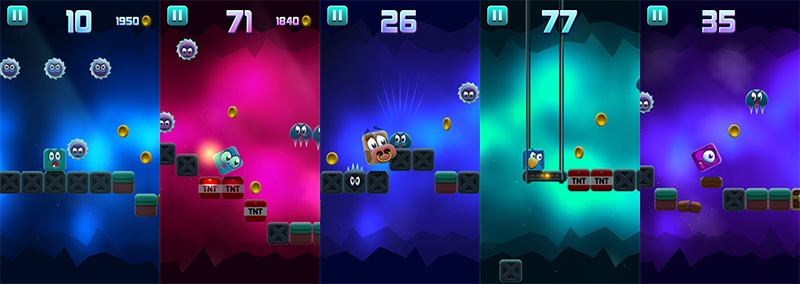# HTML5 player movement like in popular iOS game “Back to Square One” made with Phaser

One of the latest Umbrella Games hits on the App Store is Back to Square One. It’s a platform game with a single tap control scheme that’s a perfect fit for mobile devices. Roll from tile to tile, while avoiding numerous obstacles and collecting coins. Sounds easy? Think again! You’ll need to pay close attention, or you’re back to square one!Umbrella games always publishes simple but awesome games, I already blogged about Boom Dots and Down the Mountain so have a look at them too! Back to our game, it’s an horizontal endless runner, and like most of the endless runner the player actually does not run, it’s the whole environment which moves towards the player to give the illusion of movement. The basic prototype was very easy to create, the only difficult thing was handling pivot points in player movement as it’s a rotating square. Have a look at the prototype:
Click or tap on the canvas to make the player move and the terrain scroll. Have a look at the fully commented source code:
```// the game
var game;

// size of each square, in pixels
var squareSize = 40;

// square where the hero will start to walk
var startingSquare = 2;

// this is the time required to make a move, in milliseconds
var moveTime = 250;

// giving some color to ground squares
var squareColors = [0x888888, 0xaaaaaa];

// creation of the game, portrait mode 320x480
game = new Phaser.Game(320, 480);
game.state.start("PlayGame");
}

var playGame = function(game){}

playGame.prototype = {

},

create: function(){
// adding a group which will contain all terrain squares

// filling the field with squares to create the terrain
for(var i = 0; i < game.width / squareSize + 2; i++){

var square = game.add.sprite(i * squareSize, game.height / 3 * 2, "square");

// settings its anchor on the center
square.anchor.set(0.5);

// giving the square a tint color
square.tint = squareColors[i % 2];

// finally adding the square to the group
}

this.hero = game.add.sprite(startingSquare * squareSize, game.height / 3 * 2 - squareSize, "square");

// setting the anchor on its center
this.hero.anchor.set(0.5);

// flag to see if the hero can move
this.hero.canMove = true;

// input listener waiting for mouse or touch input, then calling moveSquare method
},

// this method will be called each time the player tries to move the square
moveSquare: function(){

// can the hero move?
if(this.hero.canMove){

// the hero is about to be moved so we aren't considering more inputs
this.hero.canMove = false;

// according to current square angle, we have to change its position and registration point
// in order to make it rotate along the required pivot point
// this part needs to be optimized but at the moment it works
switch(this.hero.angle){
case 0:
this.hero.x += squareSize / 2;
this.hero.y += squareSize / 2;
this.hero.pivot.x = squareSize / 2;
this.hero.pivot.y = squareSize / 2;
break;
case 90:
this.hero.x += squareSize;
this.hero.pivot.x = squareSize / 2;
this.hero.pivot.y = -squareSize / 2;
break;
case -180:
this.hero.x += squareSize;
this.hero.pivot.x = -squareSize / 2;
this.hero.pivot.y = -squareSize / 2;
break;
case -90:
this.hero.x += squareSize;
this.hero.pivot.x = -squareSize / 2;
this.hero.pivot.y = squareSize / 2;
}

// tween to scroll the terrain to the left by squareSize pixels
x: this.terrainGroup.x - squareSize
}, moveTime, Phaser.Easing.Linear.None, true);

// tween to rotate and move the hero
angle: this.hero.angle + 90,
x: this.hero.x - squareSize
}, moveTime, Phaser.Easing.Linear.None, true);

// once the tween has been completed...

// the hero can move again
this.hero.canMove = true;

// if hero's angle is zero, that is if we rotated by 360 degrees...
if(this.hero.angle == 0){

// we restore hero original pivot point and position
this.hero.pivot.x = 0;
this.hero.pivot.y = 0;
this.hero.x += squareSize / 2;
this.hero.y -= squareSize / 2;
}

// we order all terrain squares according to their x position
this.terrainGroup.sort("x", Phaser.Group.SORT_ASCENDING);

// we don't want to destroy assets to create new ones, we prefer to move the leftmost square
// which is not visible anymore to the right.
this.terrainGroup.getChildAt(0).x += this.terrainGroup.length * squareSize;

}, this);
}
}
}
```
Next time we’ll add different terrain types, coins and enemies. Meanwhile download the source code of the entire project.
214 GAME PROTOTYPES EXPLAINED WITH SOURCE CODE
// 1+2=3
// 10000000
// 2 Cars
// 2048
// Avoider
// Ballz
// Block it
// Blockage
// Bloons
// Boids
// Bombuzal
// Breakout
// Bricks
// Columns
// CubesOut
// Dots
// DROP'd
// Dudeski
// Eskiv
// Filler
// Fling
// Globe
// HookPod
// Hundreds
// InkTd
// Iromeku
// Lumines
// Magick
// MagOrMin
// Maze
// Memdot
// Nano War
// Nodes
// o:anquan
// Ononmin
// Pacco
// Phyballs
// Platform
// Poker
// Pool
// Poux
// Pudi
// qomp
// Racing
// Renju
// SameGame
// Security
// Sling
// Slingy
// Sokoban
// Splitter
// Sproing
// Stack
// Stringy
// Sudoku
// Tetris
// Threes
// Toony
// Turn
// TwinSpin
// vvvvvv
// Wordle
// Worms
// Yanga
// Zhed
// zNumbers